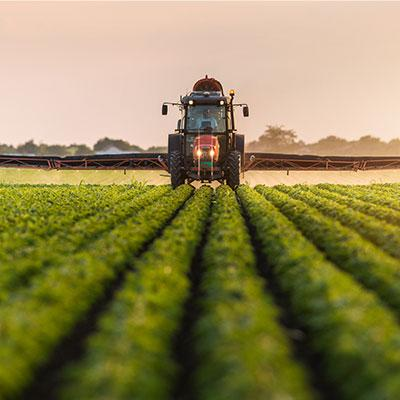# Planting of new soybean crop remains at accelerated pace in Brazil

226Porto Alegre, November 1, 2021 – The planting of the new Brazilian soybean crop once again had a good week in most of the country’s growing states in the period between November 23 and 29.

Despite some more abundant rainfall in some micro-regions of the country, the planting was satisfactory at national level, maintaining the pace above the 5-year average for this period of the year.

According to a survey carried out by SAFRAS & Mercado, until October 29, Brazilian growers had sowed 51% of the total area expected for the country in 2021/22. The index is equivalent to approximately 20,292 million hectares sown, out of the total estimate of 39.820 million hectares. In the week earlier, the index was 35.8%. In the same period of the previous year, the index reached 35%, while the five-year average for the period is 40.4%.

In Rio Grande do Sul, from an expected total area of ​​6.12 million hectares, 6% of the area were sown, or approximately 367 thousand hectares. The week before, the index was 0.5%. In the same period of the previous year, the index was 8%, while the five-year average for the period is 8.2%.

In Paraná, the planting was 63% complete of the area destined for soybeans. The index is equivalent to approximately 3.541 million hectares planted, out of a total of 5.620 million hectares estimated for the state. In the week earlier, the index was 41%. In the same period last year, the index reached 64%, while the five-year average is 65.4% for the period.

In Mato Grosso, out of a total expected area of 10.82 million hectares, 83% have already been sown, equivalent to approximately 8.981 million hectares. In the week earlier, the index was 69%. In the same period of the previous year, the index was 52%. The five-year average for the period is 67%.

In Mato Grosso do Sul, the planting reaches 67% of an estimated total area of 3.45 million hectares, equivalent to approximately 2.312 million hectares. In the week earlier, the index was 45%, while in the same period last year, the index was 50%. The five-year average for the period is 53.8%.

In Goiás, out of a total area estimated at 3.835 million hectares, 59% were sown, equivalent to approximately 2.262 million hectares. In the week earlier, the index was 37% against 35% in the same period last year. The five-year average for the period is 35.4%.

In São Paulo, out of a total area estimated at 1.185 million hectares, 65% were sown, or approximately 770 thousand hectares. In the week earlier, the index was 36%. In the same period of the previous year, the index was 19%, while the five-year average for the period is 27.6%.

In Minas Gerais, the index was at 55% of a total area estimated at 1.88 million hectares, equivalent to approximately 1.034 million hectares. In the week earlier, the index was 35%. In the same period last year, the index was 30%, while the five-year average for the period is 29.4%.

In Bahia, out of a total area estimated at 1.72 million hectares, 20% were sown, or approximately 344 thousand hectares. In the week earlier, the index was 8%, while in the same period last year the index was 8%. The five-year average for the period is 6.1%.

Weather forecasts point to a period of moisture in practically the entire country in the period between October 29 and November 4. Despite this, excessive rainfall is not expected in most states, which must allow a good planting advance.

In the period between November 5 and 11, moisture must also remain in practically the entire country, but this time with large accumulations of precipitation expected in the states of Minas Gerais, Goiás, Mato Grosso, Bahia, Maranhão, Piauí, and Pará, which must prevent better work progress.